Visitors Online: 0 | Thursday 05th December 2019

CBSE Guess > Papers > Question Papers > Class XII > 2007 > Mathematics > Delhi Set - I

Mathematics — 2007 (Set I — Delhi)

Section- A

Qs. 1. If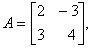show that A2 – 6A + 17 I = 0. Hence find A -1.

Qs. 2. An urn contains 7 red and 4 blue balls. Two balls are drawn at random with replacement. Find the probability of getting (a) 2 red balls (b) 2 blue balls (C) one red and one blue ball.

Qs. 3. Using properties of determinants, prove the following: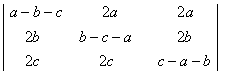= (a + b +c)3

Qs. 4. A card is drawn at random from a well-shuffled pack of 52 cards. Find the probability that it is neither an ace nor a king.

Qs. 5. Evaluate: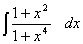Qs. 6. Solve the following differential equation:

X cos y dy = (xex log x + ex) dx

Qs. 7. Form the differential equation of the family of curves y = A cos 2x + B sin 2x, where A and B are constants.

or

Solve the following differential equation: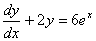Qs. 8.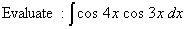Qs. 9. Using properties of definite integrals, prove the following:Qs. 10. Evalute: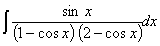Qs. 11. Find the value of k if the function.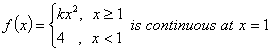Or

Evaluate: limQs. 12. Differentiate sin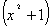with respect to x from first principal.

Qs. 13. Write the Boolean expression for the following circuit: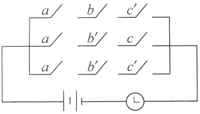Simplify the Boolean expression.

or

Show the following argument is valid: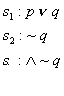Qs. 14. If y = sin, prove thatQs. 15. Verify Rolle’s theorem for the function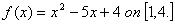Qs. 16. Using matrices ,solve the following system of equation:Qs. 17. Using integration, find the area of the region enclosed between the circle:or

EvaluateQs. 18. Find the point on the curvewhich is nearest to the point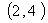or

Show that the right circular cone of least curved surface and given volume has an altitude equal to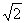times the radius of the base.

Section- B

Qs. 19. Find the projection

of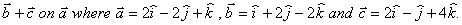Qs. 20. Find the value of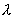which makes the vectors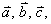coplanar, where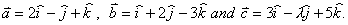Qs. 21. A particle starting with initial velocity of 30 m/sec, moves with a uniform acceleration of 9 m/sec2 Find:

1. the velocity of the particle after 6 seconds
2. how far will it go in 9 seconds.
3. its velocity when it has traversed 150 m.

Qs. 22. Find the resultant of two velocities 4 m/sec and 6 m /sec inclined to one-another at an angle of 120°.

or

A ball projected with a velocity of 28 m/sec has a horizontal range 40 m. Find the     two angles of projection.

Qs. 23. A body of weight 70 N is suspended by two strings of length 27 cm and 36 cm, fastened to two points in the same horizontal line 45 cm apart and is in equilibrium. Find the tensions in the strings.

Qs. 24. The resultant of two unlike parallel forces of 18 N and 10 N act along a line at a distance of 12 cm from the line of action of the smaller force. Find the distance between the lines of action of two forces.

Qs. 25. Find the equation of the plane which is perpendicular to the plane 5x + 3y + 6z + 8 = 0 and which contains the line of intersection of the planes x + 2y + 3z – 4 = 0 and 2x + y – z + 5 = 0.

Qs. 26. Find the equation of the sphere passing through the points (3, 0, 0), (0,-1, 0), (0, 0, -2) and having the centre on the plane 3x +2y + 4z = 1.

Section - C

Qs. 27. Find the face value of a bill, discounted at 6% per annum 146 days before the due date, if the banker’s gain is Rs. 36.

Qs. 28. A bill for Rs. 7650 was drawn on 8th March 2005 at 7 months. It was discounted on 18 May 2005 and the holder of the bill received Rs. 7497. What rate of interest did the banker charge?

Qs. 29. There are two bags I and II. Bag I contains 2 white and 3 red balls and Bag II contains 4 white and 5 red balls. One ball is drawn at random from one of the bags and is found to be red. Find the probability that it was drawn from bag II.

Qs. 30. Find mean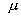variance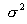for the following probability distribution:

 X 0 1 2 3 P(X)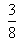Or

Find the binomial distribution for which the mean is 4 and variance 3

Qs. 31. A, B, C entered into a partnership investing Rs. 12000, Rs. 16000 and Rs. 20000 respectively. A as working partner gets 10% of the annual profit for the same. After 5 months, B invested Rs. 2000 more while C withdrew Rs. 2000 after 8 months from the start of the business. Find the share of each in an annual profit of Rs. 97000.

Qs. 32. Find present value of an annuity due of Rs. 700 per annum payable at the beginning of each year for 2 years allowing interest 6% per annum, compounded annually.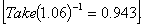Qs. 33. The total cost .C(x), associated with the production and making x units of an item given by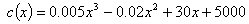Find (i) the average cost function (ii) the average cost of output of 10 units (iii) the marginal cost function (iv) the marginal cost when 3 units are produced.

Qs. 34. If a young man rides his motorcycle at 25km/hour, he had to spend Rs. 2 per km on petrol. If he rides at a faster speed of 40 km/hour, the petrol cost increases at Rs. 5 per km. He has Rs. 100 to spend on petrol and wishes to find what is the maximum distance he can travel within one hour. Express this as an LPP and solve it graphically.

 Mathematics 2007 Question Papers Class XII DelhiSet I Set III

CBSE 2007 Question Papers Class XII